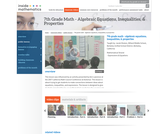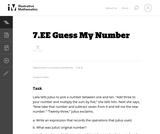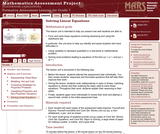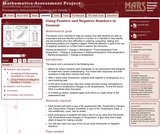Updating search results...

# 5 Results

View
Selected filters:
• MCCRS.Math.Content.7.EE.B.4Rating
0.0 stars

This lesson is about trying to get students to make connections between ideas about equations, inequalities, and expressions. The lesson is designed to give students opportunities to use mathematical vocabulary for a purpose to describe, discuss, and work with these symbol strings.The idea is for students to start gathering global information by looking at the whole number string rather than thinking only about individual procedures or steps. Hopefully students will begin to see the symbol strings as mathematical objects with their own unique set of attributes. (7th Grade Math)

Subject:
Algebra
Numbers and Operations
Material Type:
Activity/Lab
Lecture
Lesson Plan
Teaching/Learning Strategy
Provider:
Noyce Foundation
Provider Set:
Inside Mathematics
Author:
Disston, Jacob
11/30/2011Only Sharing Permitted
CC BY-NC-ND
Rating
4.5 stars

This lesson unit is intended to help assess how well students are able to interpret and use scale drawings to plan a garden layout. This involves using proportional reasoning and metric units.

Subject:
Algebra
Geometry
Ratios and Proportions
Material Type:
Assessment
Lesson Plan
Provider:
Shell Center for Mathematical Education
Provider Set:
Mathematics Assessment Project (MAP)
04/26/2013Unrestricted Use
CC BY
Rating
0.0 stars

This problem asks the students to represent a sequence of operations using an expression and then to write and solve simple equations. The problem is posed as a game and allows the students to visualize mathematical operations. It would make sense to actually play a similar game in pairs first and then ask the students to record the operations to figure out each other's numbers.

Subject:
Mathematics
Algebra
Material Type:
Activity/Lab
Provider:
Illustrative Mathematics
Provider Set:
Illustrative Mathematics
Author:
Illustrative Mathematics
08/07/2012Only Sharing Permitted
CC BY-NC-ND
Rating
5.0 stars

This lesson unit is intended to help teachers assess how well students are able to: form and solve linear equations involving factorizing and using the distributive law. In particular, this unit aims to help teachers identify and assist students who have difficulties in: using variables to represent quantities in a real-world or mathematical problem and solving word problems leading to equations of the form px + q = r and p(x + q) = r.

Subject:
Algebra
Material Type:
Assessment
Lesson Plan
Provider:
Shell Center for Mathematical Education
Provider Set:
Mathematics Assessment Project (MAP)
04/26/2013Only Sharing Permitted
CC BY-NC-ND
Rating
3.5 stars

This lesson unit is intended to help teachers assess how well students are able to understand and use directed numbers in context. It is intended to help identify and aid students who have difficulties in ordering, comparing, adding, and subtracting positive and negative integers. Particular attention is paid to the use of negative numbers on number lines to explore the structures: starting temperature + change in temperature = final temperature final temperature Đ change in temperature = starting temperature final temperature Đ starting temperature = change in temperature.

Subject:
Numbers and Operations
Material Type:
Assessment
Lesson Plan
Provider:
Shell Center for Mathematical Education
Provider Set:
Mathematics Assessment Project (MAP)